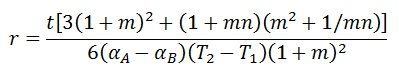# Bimetallic Thermometer

Definition: The bimetallic thermometer uses the bimetallic strip which converts the temperature into the mechanical displacement. The working of the bimetallic strip depends on the thermal expansion property of the metal. The thermal expansion is the tendency of metal in which the volume of metal changes with the variation in temperature.

Every metal has a different temperature coefficient. The temperature coefficient shows the relation between the change in the physical dimension of metal and the temperature that causes it. The expansion or contraction of metal depends on the temperature coefficient, i.e., at the same temperature the metals have different changes in the physical dimension.

##Working Principle of Bimetallic Thermometer

The working principle of bimetallic thermometer depends on the two fundamental properties of the metal.

1. The metal has the property of thermal expansion, i.e., the metal expand and contract concerning the temperature.
2. The temperature coefficient of all the metal is not same. The expansion or contraction of metals is different at the same temperature.

## Constructions of Bimetallic Thermometer

The bimetallic strip is constructed by bonding together the two thin strips of different metals. The metals are joined together at one end with the help of the welding. The bonding is kept in such a way that there is no relative motion between the two metals. The physical dimension of the metals varies with the variation in temperature.

Since the bimetallic strip of the thermometer is constructed with different metals. Thereby, the length of metals changes at different rates. When the temperature increases, the strip bends towards the metal which has a low-temperature coefficient. And when the temperature decreases, the strip bends towards the metal which has a high-temperature coefficient.

The figure below shows the bimetallic strip in the form of the straight cantilever beam. The strip fixed at one end and deflects at the other end.The range of deflection of bimetallic strip depends on the type of metals used for construction. The deflection of the metal is directly proportional to the length of the strip and the variation of temperature and is inversely proportional to the thickness of the strips.Let understands this with the help of the mathematical formula. Consider the bimetallic strip is made of two different metals, i.e., metal A and metal B. Both the metals have a different temperature coefficient. The T2 – T1 shows the variation of temperature, which causes the expansion of the string.Where, t – the total thickness of the strip
n – the ratio of moduli of elasticity = EB/EA
m – the ratio of the thickness
T2 – T1 – change in temperature
tA, tB – the thickness of metal A and metal B.
αA, αB– the thermal coefficient of expansion of metal A and B.

The expansion causes the strip to move in the uniform circular arc. The radius of the arm is given by the formula shown below.The above equation shows that the strip bend towards the metals which has a low-temperature coefficient (when the temperature increases) and the inverse will happen when the temperature decreases.

For practical applications, the strip is made of metals whose moduli of elasticity and thickness are same. The moduli of elasticity show the ability of the material to regain its original position or shape after the removal of force or load.

Consider the strip is fixed at one end and free to move at the other end. When the temperature surroundings the strip vary, the strip bends towards the circular arc.

Consider the strip is made of the metal having thickness t/2.

So,If one of the metals has the very small temperature coefficient, then theAbove equation shows that if one end of the metal is fixed, the deflection of the free end of metals shows the variation of temperature. This thermometer is not used for industrial application because of low sensitivity and small deflection. The sensitivity of the thermometer increases with the increase of the length of the strip.

### Types of Bimetallic Strip

The linear strip shows the small deflection. If the length of strip increases, the size of thermometer also increases. For keeping the size of the thermometer in the manageable limit, the helix or spiral strip is used for making the thermometer.

Spiral Strip bimetallic thermometer – In bimetallic strip thermometer, the spiral-shaped strip is used. This type of thermometer is used for measuring the ambient temperature. Because of the thermal expansion property of metal the deformation occurs in the spring with the variation of temperature. The pointer and dials attached to the spring, which indicates the variation of temperature.Helical Types Bimetallic Strip – The helix type bimetallic strip is mostly used for industrial applications. In this thermometer, the helix shape strip is used for measuring the temperature. The free end of the strip is connected to the pointer. The deflection of the strip shows the variation of temperature.

The thermometer is simple in construction, robust and less expensive.